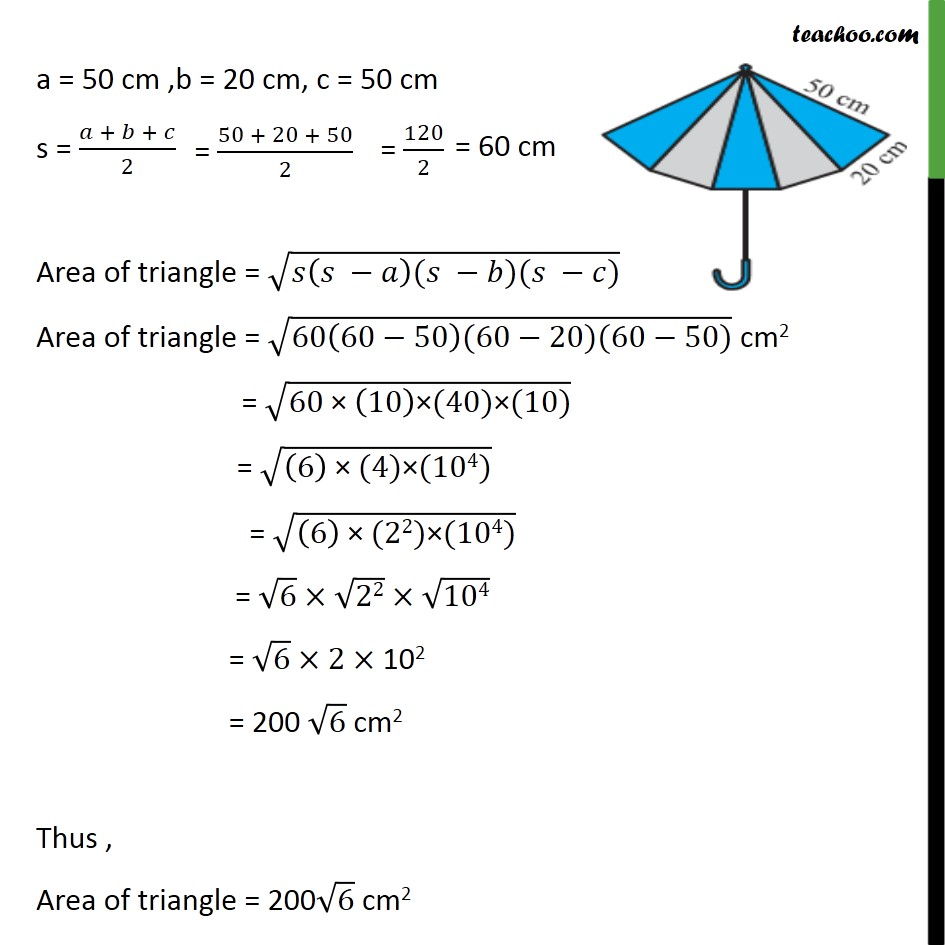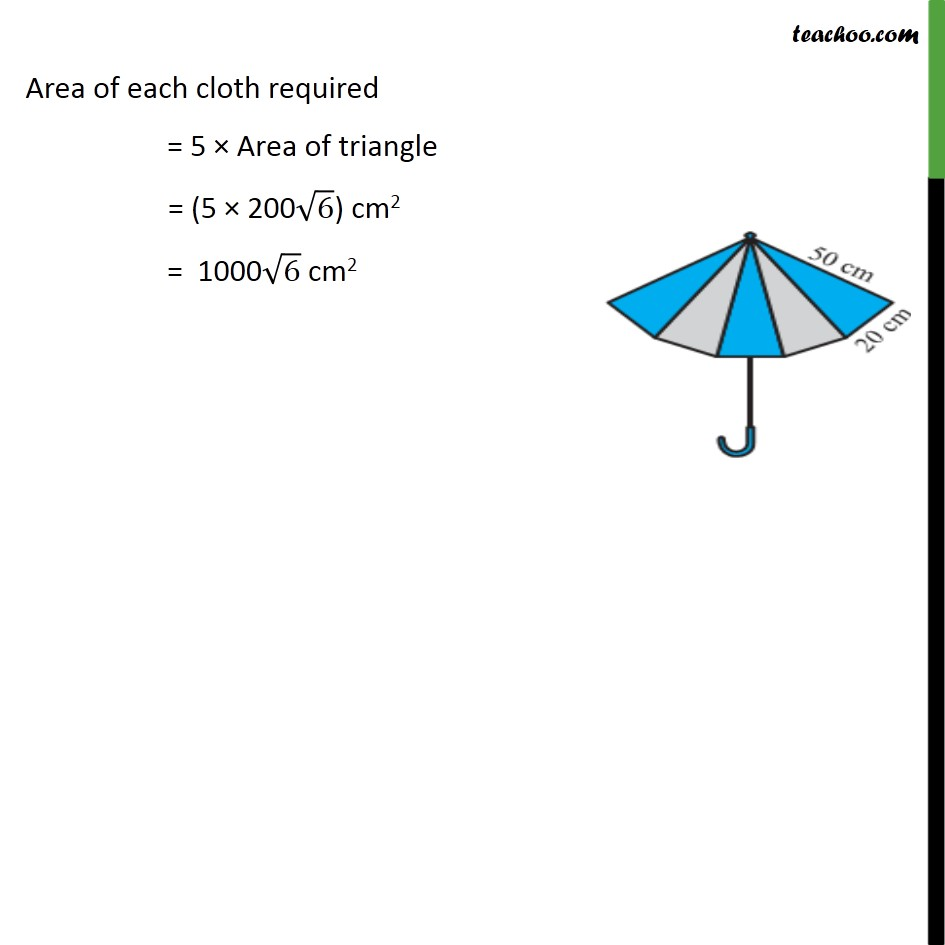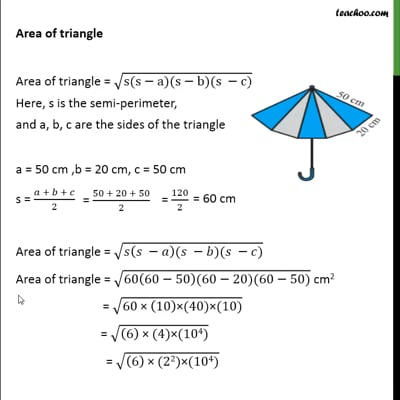Ex 12.2

Chapter 12 Class 9 Herons Formula (Term 1)
Serial order wiseThis video is only available for Teachoo black users

### Transcript

Ex 12.2, 6 An umbrella is made by stitching 10 triangular pieces of cloth of two different colours (see the given figure), each piece measuring 20 cm, 50 cm and 50 cm. How much cloth of each colour is required for the umbrella? There are 10 triangles, 5 triangles of blue color and 5 triangles of grey color Area of each cloth = 5 × (Area of 1 triangle) Area of triangle Area of triangle = √(s(s−a)(s−b)(s −c)) Here, s is the semi-perimeter, and a, b, c are the sides of the triangle a = 50 cm ,b = 20 cm, c = 50 cm s = (𝑎 + 𝑏 + 𝑐)/2 Area of triangle = √(𝑠(𝑠 −𝑎)(𝑠 −𝑏)(𝑠 −𝑐)) Area of triangle = √(60(60−50)(60−20)(60−50)) cm2 = √(60" × " (10)"×" (40)"×" (10)) = √((6)" ×" (4)"×" (104)) = √((6)" ×" (22)"×" (104)) = √6 ×√22× √104 = √6 ×2× 102 = 200 √6 cm2 Thus , Area of triangle = 200√6 cm2 Area of each cloth required = 5 × Area of triangle = (5 × 200√6) cm2 = 1000√6 cm2